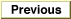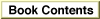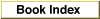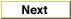Legacy DocumentImportant: The information in this document is obsolete and should not be used for new development.

Inside Macintosh: QuickDraw GX Environment and Utilities /
Chapter 8 - QuickDraw GX Mathematics

Summary of QuickDraw GX Mathematics

Constants and Data Types

Number Formats and Constants

typedef long fract;
typedef unsigned short gxColorValue;
struct gxPolar {
Fixed angle;
};
struct wide {
long hi;
unsigned long lo;
};
#define fixed1             ((Fixed) 0x00010000)        /* = 1.0 for Fixed */
#define fract1             ((fract) 0x40000000)        /* = 1.0 for fract */
#define gxColorValue1      ((gxColorValue) 0xFFFF) /* 1.0 for gxColorValue*/
#define gxPositiveInfinity ((Fixed) 0x7FFFFFFF)    /* for Fixed and fract */
#define gxNegativeInfinity ((Fixed) 0x80000000)    /* for Fixed and fract */

The Mapping Structure

struct gxMapping {
Fixed map;
};

Number-Conversion Macros

Format Conversions

#define FixedToFract(a)	((fract) (a) << 14)
#define FractToFixed(a)	((Fixed) (a) + 8192L >> 14)
#define FixedToInt(a)	((short) ((Fixed) (a) + fixed1/2 >> 16))
#define IntToFixed(a)	((Fixed)(a) << 16)
#define ff(a)		((Fixed)(a) << 16)
#define FixedToFloat(a)	((float)(a) / fixed1)
#define FloatToFixed(a)	((Fixed)((float) (a) * fixed1))
#define fl(a)		((Fixed)((float) (a) * fixed1))
#define FractToFloat(a)	((float)(a)/fract1)
#define FloatToFract(a)	((fract)((float)(a)*fract1))
#define ColorToFract(a)	(((fract)(a)<<14) + ((fract)(a) +2 >>2))
#define FractToColor(a)	((gxColorValue)((a)-((a)>>16)+8191>>14))

Rounding, Truncating, and Square Root Operations

#define FixedRound(a)		((short) ((Fixed)(a) + fixed1/2 >> 16))
#define FixedTruncate(a)	((short)((Fixed)(a) >> 16))
#define FixedSquareRoot(a)	((Fixed)FractSquareRoot(a) + 64 >>7)

Mathematical Functions

Fixed-Point Operations

Fixed FixedMultiply	(Fixed multiplicand, Fixed multiplier);
Fixed FixedDivide	(Fixed dividend, Fixed divisor);
long MultiplyDivide	(long multiplicand, long multiplier,
long divisor);
unsigned long Magnitude	(long deltaX, long deltaY);
fract FractSineCosine	(Fixed degrees, fract *cosine);
fract FractSquareRoot	(fract source);
fract FractCubeRoot	(fract source);
fract FractMultiply	(fract multiplicand, fract multiplier);
fract FractDivide	(fract dividend,fract divisor);

Operations on wide Numbers

wide *WideAdd		(wide *target, const wide *source);
wide *WideSubtract	(wide *target, const wide *source);
wide *WideNegate	(wide *target);
wide *WideShift		(wide *target, long shift);
wide *WideMultiply	(long multiplicand, long multiplier,
wide *target);
long WideDivide		(const wide *dividend, long divisor,
long *remainder);
wide *WideWideDivide	(wide *dividend, long divisor, long *remainder);
unsigned long WideSquareRoot
(const wide *source);
short WideScale		(const wide *w);
short WideCompare	(const wide *target, const wide *source);

Vector Operations

wide *VectorMultiply	(long count, const long *vector1, long step1,
const long *vector2, long step2, wide *dot);
long *VectorMultiplyDivide(long count, const long *vector1, long step1,
const long *vector2, long step2, long divisor);

Cartesian and Polar Coordinate Point Conversions

gxPoint *PolarToPoint	(const gxPolar *ra, gxPoint *xy);
gxPolar *PointToPolar	(const gxPoint *xy, gxPolar *ra);

Random Number Generation

unsigned long RandomBits(long count, long focus);
void SetRandomSeed	(const wide *seed);
wide *GetRandomSeed	(wide *seed);

long LinearRoot		(Fixed first, Fixed last, fract t[]);
long QuadraticRoot	(Fixed first, Fixed control, Fixed last,
fract t[]);

Bit Analysis

short FirstBit		(unsigned long x);

Mapping Functions

Manipulating and Applying Mappings

gxMapping *CopyToMapping	(gxMapping *target, const gxMapping *source);
gxMapping *NormalizeMapping	(gxMapping *target);
gxMapping *ResetMapping		(gxMapping *target);
gxMapping *InvertMapping	(gxMapping *target, const gxMapping *source);
gxMapping *MapMapping		(gxMapping *target, const gxMapping *source);
void MapPoints			(const gxMapping source, long count,
gxPoint vector[]);

Modifying Mappings

gxMapping *MoveMapping		(gxMapping *target,
Fixed hOffset, Fixed vOffset);
gxMapping *MoveMappingTo	(gxMapping *target,
Fixed hPosition, Fixed vPosition);
gxMapping *ScaleMapping		(gxMapping *target,
Fixed hFactor, Fixed vFactor,
Fixed xCenter, Fixed yCenter);
gxMapping *RotateMapping	(gxMapping *target, Fixed angle,
Fixed xCenter, Fixed yCenter);
gxMapping *SkewMapping		(gxMapping target, Fixed skewX, Fixed skewY,
Fixed xCenter, Fixed yCenter);